# Thermometer

The thermometer showed -12 degrees Celsius in the morning then the temperature rises by 4 degrees and later again increased by 2 degrees at the evening has fallen by 5 degrees and then falls 3 degrees. What end temperature does the thermometer show?

t =  -14 °C

### Step-by-step explanation:

$t=-12+4+2-5-3=-14\text{°C}$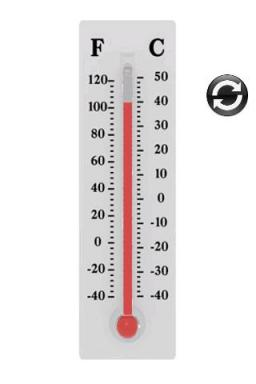Did you find an error or inaccuracy? Feel free to write us. Thank you!## Related math problems and questions:

• Thermometer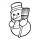The thermometer showed -3°C in the morning. Then the temperature was increased by 1°C again increased by 1°C and then decreased by 1°C and then decreased by 4°C. Which terminal temperature thermometer shows?
• Now isNow is 5°C morning. What will the temperature be if i. It rises by 12°C ii. It falls by 15°C
• Temperature up and dowm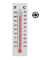At 6 AM, the temperature is 19.4°C. Between 6 AM and noon, the temperature rises 3.8°C. Between noon and 6 PM, the temperature falls 2.5°C. What is the temperature at 6 PM?
• This morningThis morning it was 80 degrees F outside the temperature increased 12 degrees F. What is the temperature now?
• Temperature variationsToday's temperature was 80 degrees, and then the temperature dropped 10 degrees. Then it dropped 15 degrees again, then the next day, the temperature went up 2 degrees. What would the temperature be?
• Temperature 13Work out the temperature after each change. The temperature starts at 6°C and falls by 13°C. The temperature starts at 2°C and falls by 8°C
• The temperature 10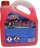The temperature in a freezer is -15°C, and it increases by 3°C.
• Lower temperatureThe level of temperature of the liquid in a thermometer is 24.32°C lower than the boiling point of water. What is the thermometer reading? Show your answer by drawing and shading the thermometer.
• The level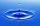The level of temperature of the liquid in a thermometer is 24.32°C lower than the boiling point of water. What is the thermometer reading? Show your answer by drawing and shading the thermometer.
• Warmer weatherThis morning it was -6 °C. What temperature did the thermometer show yesterday if it was three times warmer?
• The temperature 8The temperature on Monday was –6°C. On Tuesday, the temperature was 3 degrees lower. Write down the temperature on Tuesday.
• OpheliaOphelia recorded the temperature of a cold store every two hours. 1. At 6 am, it was -4°C, and at 8 am, it was -1°C. By how much did the temperature rise? 2. The temperature went up by 5°C in the next two hours. .What was the temperature at 10 am?
• Celsius 25 degrees Celsius at midday dropped 12°Celsius degree by evening. What is the temperature?
• The temperature 9The temperature at 9 AM is 2 degrees. The temperature rises 3 more degrees by noon. Which expression describes the temperature at noon? Calculate the new temperature value.
• At midmorningThe temperature at midmorning was 31°C. Early in the evening, the temperature dropped by 5°C. What was the resulting temperature?
• Change in temperatureStarting temperature is 21°C, the highest temperature is 32°C. What is the change in temperature?
• Freezer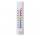The temperature is -8 degrees Celsius in the freezer. The outside temperature is 2 degrees Celsius. One minute took to change the temperature by one degree Celsius if we open the door. How many minutes the temperature in the freezer will rise?